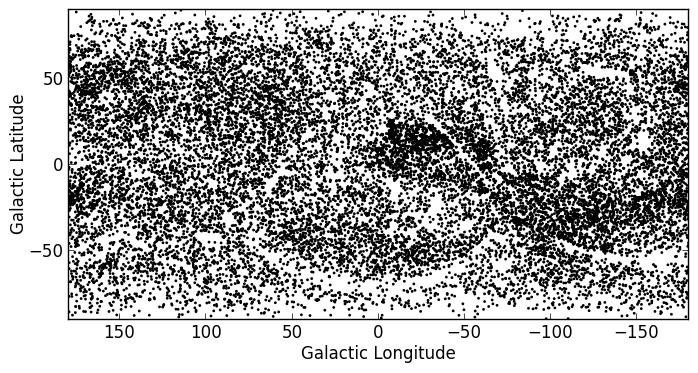#### Previous topic

Unit manipulation

# Celestial Coordinates¶

## Representing and converting coordinates¶

Astropy includes a framework to represent celestial coordinates and transform between them. Astropy 0.2 only includes a few common coordinate systems (ICRS, FK4, FK5, and Galactic), but future versions will include more built-in coordinate systems, and users can already define their own systems. In addition, while the current version only supports transformation of individual scalar coordinates, arrays will be supported in future.

Coordinate objects are instantiated with a flexible and natural approach that supports both numeric angle values and (limited) string parsing:

```>>> from astropy import coordinates as coord
>>> from astropy import units as u
>>> coord.ICRSCoordinates(ra=10.68458, dec=41.26917, unit=(u.degree, u.degree))
<ICRSCoordinates RA=10.68458 deg, Dec=41.26917 deg>
>>> coord.ICRSCoordinates('00h42m44.3s +41d16m9s')
<ICRSCoordinates RA=10.68458 deg, Dec=41.26917 deg>
```

The individual components of a coordinate are Angle objects, and their values are accessed using special attributes:

```>>> c = coord.ICRSCoordinates(ra=10.68458, dec=41.26917, unit=(u.degree, u.degree))
>>> c.ra
<RA 10.68458 deg>
>>> c.ra.hours
0.7123053333333333
>>> c.ra.hms
(0.0, 42, 44.2992000000001)
>>> c.dec
<Dec 41.26917 deg>
0.7202828960652683
```

To convert to some other coordinate system, the easiest method is to use attribute-style access with short names for the built-in systems:

```>>> c.galactic
<GalacticCoordinates l=121.17422 deg, b=-21.57283 deg>
```

but explicit transformations via the transform_to method are also available:

```>>> c.transform_to(coord.GalacticCoordinates)
<GalacticCoordinates l=121.17422 deg, b=-21.57283 deg>
```

The astropy.coordinates subpackage also provides a quick way to get coordinates for named objects (with an internet connection). All coordinate classes have a special class method, from_name(), that accepts a string and queries Sesame to retrieve coordinates for that object:

```>>> c_eq = coord.ICRSCoordinates.from_name("M16")
>>> c_eq
<ICRSCoordinates RA=274.70000 deg, Dec=-13.80670 deg>
```

This works for any coordinate class:

```>>> c_gal = coord.GalacticCoordinates.from_name("M16")
>>> c_gal
<GalacticCoordinates l=16.95408 deg, b=0.79335 deg>
```

Note

This is intended to be a convenience, and is very simple. If you need precise coordinates for an object you should find the appropriate reference for that measurement and input the coordinates manually.

## Practical Exercises¶

Level 1

Find the coordinates of the Crab Nebula in ICRS coordinates, and convert them to Galactic Coordinates

Click to Show/Hide Solution

```>>> from astropy import coordinates as coord
>>> crab = coord.ICRSCoordinates.from_name('M1')
>>> print crab
<ICRSCoordinates RA=83.63308 deg, Dec=22.01450 deg>
>>> crab_gal = crab.transform_to(coord.GalacticCoordinates)
>>> print crab_gal
<GalacticCoordinates l=-175.44248 deg, b=-5.78434 deg>
```

Level 2

Using the ROSAT Point source catalog (from Tabular data), convert all the equatorial coordinates to Galactic coordinates, and make a new plot (but don’t worry about the bright sources).

Click to Show/Hide Solution

```from astropy import units as u
from astropy import coordinates as coord
from astropy.table import Table
from matplotlib import pyplot as plt

l = []
b = []
for row in t:
eq = coord.FK5Coordinates(row['RAJ2000'], row['DEJ2000'], unit=(u.degree, u.degree))
gal = eq.transform_to(coord.GalacticCoordinates)
l.append(gal.l.degrees)
b.append(gal.b.degrees)

fig = plt.figure()
ax.scatter(l, b, s=1, color='black')
ax.set_xlim(180., -180.)
ax.set_ylim(-90., 90.)
ax.set_xlabel("Galactic Longitude")
ax.set_ylabel("Galactic Latitude")

fig.savefig('coord_level2.png', bbox_inches='tight')
```Level 3

Try and make a plot similar to this!

Copyright: Smithsonian Astrophysical Observatory under terms of CC Attribution 3.0